Function Repository Resource:

# PossiblyDifferentiableQ

Determine whether a single-variable, real-valued function is differentiable

Contributed by: Wolfram|Alpha Math Team
 ResourceFunction["PossiblyDifferentiableQ"][f,x] returns True if f is differentiable with respect to x for all real numbers. ResourceFunction["PossiblyDifferentiableQ"][f,{x,n}] returns True if f is n-times differentiable with respect to x for all real numbers. ResourceFunction["PossiblyDifferentiableQ"][{f,cons},x] returns True if f is differentiable with respect to x for the real numbers that satisfy the constraint cons. ResourceFunction["PossiblyDifferentiableQ"][{f,cons},{x,n}] returns True if f is n-times differentiable with respect to x for the real numbers that satisfy the constraint cons.

## Details

ResourceFunction["PossiblyDifferentiableQ"] has the attribute HoldFirst.
ResourceFunction["PossiblyDifferentiableQ"] takes only real-valued functions.
The constraint cons can be any logical combination of equations or inequalities:
 lhs==rhs equations lhs>rhs,lhs≥rhs,lhs

## Examples

### Basic Examples (1)

Determine if a function is differentiable over all real numbers:

 In:=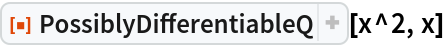Out=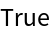In:=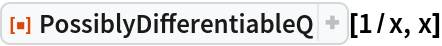Out=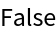### Scope (3)

Determine if a function is differentiable for a particular set of x-values:

 In:=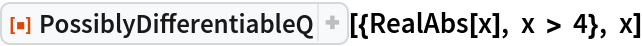Out=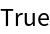The constraint can also be a logical combination of inequalities:

 In:=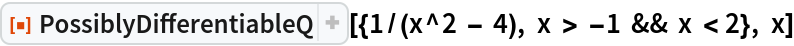Out=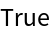Determine if a function is 5-times differentiable:

 In:=Out=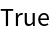### Properties and Relations (1)

PossiblyDifferentiableQ has the attribute HoldFirst, enabling calculations such as the following:

 In:=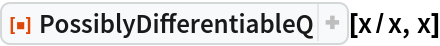Out=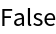### Possible Issues (2)

For some edge cases, PossiblyDifferentiableQ will return True even if the given function is not differentiable everywhere:

 In:=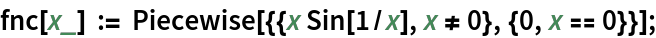In:=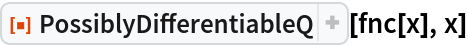Out=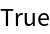Since PossiblyDifferentiableQ takes only real-valued functions, it will treat Abs as RealAbs:

 In:=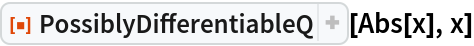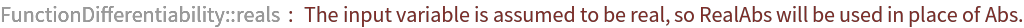Out=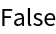## Publisher

Wolfram|Alpha Math Team

## Version History

• 2.0.0 – 23 March 2023
• 1.0.0 – 04 January 2022

## Author Notes

To view the full source code for PossiblyDifferentiableQ, evaluate the following:

 In:=